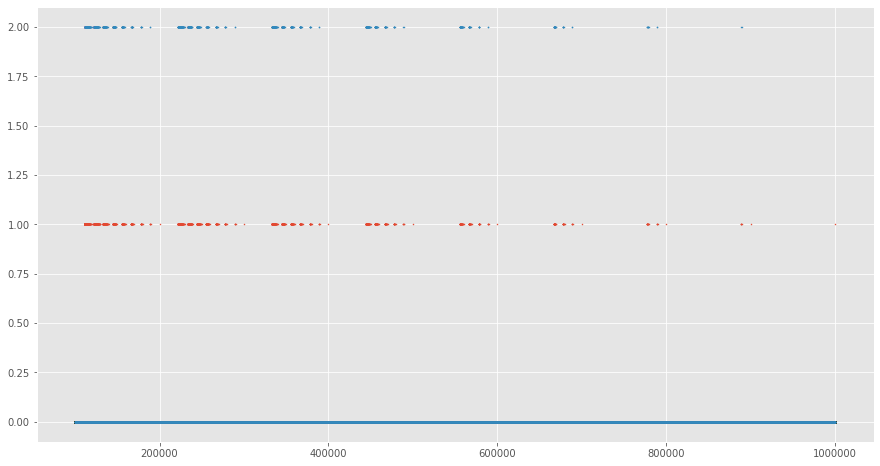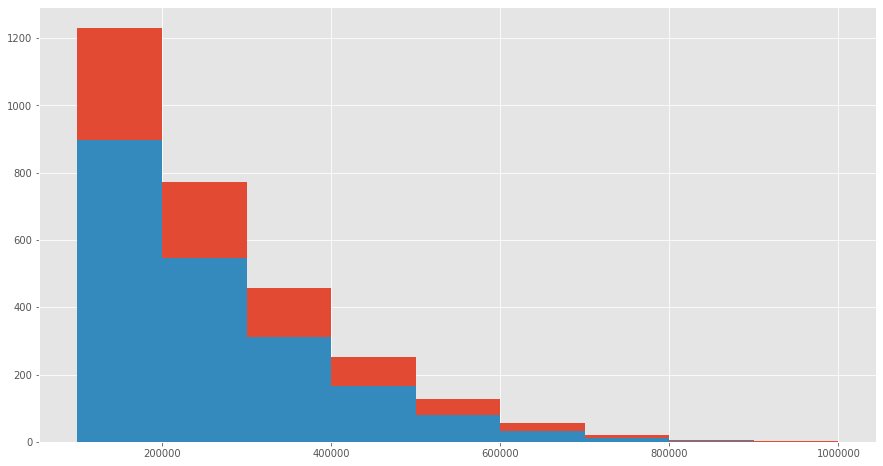# Advent of Code Day 4¶

It is a six-digit number. The value is within the range given in your puzzle input. Two adjacent digits are the same (like 22 in 122345). Going from left to right, the digits never decrease; they only ever increase or stay the same (like 111123 or 135679).

111111 meets these criteria (double 11, never decreases). 223450 does not meet these criteria (decreasing pair of digits 50). 123789 does not meet these criteria (no double).

How many different passwords within the range given in your puzzle input meet these criteria?

In :
test_input = [193651, 649729 + 1]

In :
def iter_len(it):
return sum(1 for _ in it)

In :
def adjacent_same(x):
return any(a == b for a,b in zip(x, x[1:]))

def increasing(x):
return all(int(a) <= int(b) for a, b in zip(x, x[1:]))


## Part 1¶

In :
%time iter_len(x for x in range(*test_input) if adjacent_same(str(x)) and increasing(str(x)))

CPU times: user 1.09 s, sys: 3.24 ms, total: 1.09 s
Wall time: 1.1 s

Out:
1605

## Part 2¶

An Elf just remembered one more important detail: the two adjacent matching digits are not part of a larger group of matching digits.

Given this additional criterion, but still ignoring the range rule, the following are now true:

112233 meets these criteria because the digits never decrease and all repeated digits are exactly two digits long. 123444 no longer meets the criteria (the repeated 44 is part of a larger group of 444). 111122 meets the criteria (even though 1 is repeated more than twice, it still contains a double 22). How many different passwords within the range given in your puzzle input meet all of the criteria?

In :
import re

digits = [a for a,b in zip(x, x[1:]) if a == b]
return any(len(re.findall(a, x)) == 2 for a in digits)

In :
%time iter_len(x for x in range(*test_input) if limited_adjacent_same(str(x)) and increasing(str(x)))

CPU times: user 1.84 s, sys: 91 µs, total: 1.84 s
Wall time: 1.84 s

Out:
1102
In :
for x in map(str, [111111, 223450, 123789]):

111111 True True False
223450 False True True
123789 True False False

In :
xs = list(range(100000, 1000000))
ys = [adjacent_same(str(x)) and increasing(str(x)) for x in xs]
zs = [limited_adjacent_same(str(x)) and increasing(str(x)) for x in xs]

In :
from matplotlib import pyplot as plt
%matplotlib inline
plt.style.use('ggplot')
plt.figure(figsize=(15, 8))
plt.scatter(xs, ys, s=1)
plt.scatter(xs, [2 * z for z in zs], s=1)
plt.show()In :
xs = list(range(100000, 1000000))
ys = [x for x in xs if adjacent_same(str(x)) and increasing(str(x))]
zs = [x for x in xs if limited_adjacent_same(str(x)) and increasing(str(x))]

In :
plt.figure(figsize=(15, 8))
plt.hist(ys, bins=9, range=(100000, 1000000))
plt.hist(zs, bins=9, range=(100000, 1000000))
plt.show()7 minutes for part 1, 14min 49s total